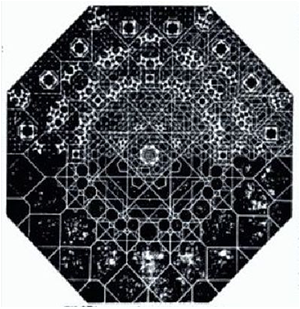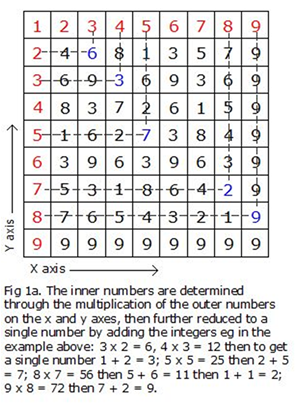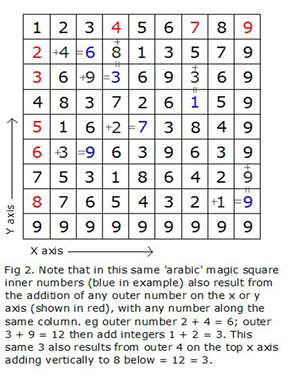# The Ant 1000 Divine Cube – 1/7

### The Ant (1000 Number) Divine Cube

Created by ANTHONY JOHN WILLIAMS (born 03 December 1951, Durban, South Africa)
Note; This is in 7 sections. For my shorter version refer Graham Hancock website under articles or the link as follows:- https://grahamhancock.com/williamsa1/

### Discovery introduction.

I had my ‘eureka’ moment back in 1985 but have not revealed this discovery until now. I always strongly felt that this discovery must have relevance, but never knew where to go with it. Based on my original curiosity regarding the two dimensional ‘Arabic’ Magic Square, I have discovered the inherent form of these number patterns within a three dimensional space, thus creating the thousand number ‘cube’.

I have discovered a hidden and ‘inherent potential’ underlying the physical pattern for the numbers 1 to 9, from the two dimensional layout of the ancient Arabic Magic Square and into the realm of three dimensions, creating a ‘cube’ made up of 1000 (10 x 10 x 10) smaller number ‘cubes’. Each smaller ‘cube’ represents a number and each number mathematically forms a ‘regular’ pattern in both two and three dimensions, on both the faces and throughout the inner heart of this cube. Each of these numbers (1 to 9) has a resultant pattern which works through the full depth of the discovered number cube from each of the six sides, changing pattern at each level, and eventually turning 90 degrees to arrive at the opposite face. There is a type of hidden ‘Universal Truth’ in the logic of the layout of the numbers 1 to 9, which is made physically apparent in this cube. The overall mathematics, the quantity of number sequences and number pattern relationships within the cube are too numerous for me to unravel, but I have explored quite a few. I intend to call this overall cube of 1000 number cubes the Ant (1000 Number) Cube

The convincing importance of this discovery re-awoke in me when I more recently read the book ‘Heavens Mirror – Quest for the Lost Civilisation’ by Graham Hancock and Santha Faiia, and the books ‘Thoth Architect of the Universe’ and ‘K2’ by Ralph Ellis and saw clear links between the number patterns revealed in the Ant Cube and the ancient sacred number sequences, relationships and mysteries described in parts of these books .

The initial inspiration came to me in 1978 when I read through a most interesting 1973 book titled ‘Shelter’ edited by Lloyd Kahn and came across the fascinating article by David Saltman on ‘Decoding Arabic Design’. David Saltman described the nine by nine ‘arabic’ magic number square, passed down through time and attributed to the Persians. He mentioned that the Persians linked equal numbers in this square with straight lines and used the resulting pattern to create the design for the complex and beautifully intricate domed and arched ceilings in their palaces and places of worship. Note that this is from the two dimensional square of the numbers 1 to 9. From what I now understand, the underlying mathematics shown in this two dimensional number square may link back to a far more ancient knowledge. Was the Three Dimensional potential (i.e. my Ant Cube discovery) from this same square perhaps also known in ancient times?

### Examining the ‘Arabic’ Magic Square

I will start by briefly describing D Saltman’s Arabic Magic Square as outlined in the book ‘Shelter’ mentioned above. This is a ‘simple’ grid square, numbered 1 to 9, left to right, along the top X axis, and 1 to 9, top to bottom, along the left hand side Y axis. The number 1, in the top left corner is common to both axes. From the layout of numbers shown in this square, I understood or where not explained in this book “Shelter’, was able to deduce the following: i.e.

The numbers placed in the remainder of the square are created as a multiple of these two rows of X and Y numbers where they intersect. Furthermore these resultant numbers, if greater than one digit, are added together to result in a single number for example 2 x 2 = 4 (single digit so remains as a 4); 5 x 2 = 10 but to get a single number 10 = 1 + 0 = 1; 6 x 2 = 12 which in turn = 1 + 2 = 3;  9 x 2 = 18 = 1 + 8 = 9, etc. Note that 9 multiplied by any number results in a final single number 9, hence the number 9 forms the outer two sides as can be seen on the image below (Fig 1a) of the ‘Arabic’ Magic Square.Fig 1. Alhambra Dome design. Apparently the ‘designer’ would create a net by connecting lines from number patterns, eg all sixes in the magic square, and then would overlay several such number nets at different angles to create intricate patterns as the inspiration for such arabic design works.Fig 1a
Furthermore, with reference to Fig 2 below, any of the outer X or Y numbers 1 to 8 (exclude the perimeter 9’s for clarity at this stage), added to any number within that same number ‘column’ or ‘line’ within the square, results in the next or following number in that column. For example:  the outer 2 (left hand side or Y axis) added to the next horizontal number (along X axis), which is 4, results in 2 + 4 = 6. The outer 3 (Y axis) added to the third horizontal number (along the X axis) which is 9, results in 3 + 9 = 12, which in turn to obtain a single digit 12 = 1 + 2 = 3. Or the top outer number 7 (X axis) added to the third number vertically down that same column, which is the number 3, results in the fourth number down being 7 + 3 = 10 = 1 + 0 = 1. Or the top outer number 9 (X axis) added to the seventh number vertically down that same column, which is 9, = 18 which in turn 1 + 8 = 9.

It is interesting to note that all numbers in the square relate to being the result of the multiplication of the outer numbers on the X and Y axes, but also as the addition of any of the same outer numbers on the X axis or Y axis with the previous number within the same column within the square, as shown in Fig 2 below.Fig 2